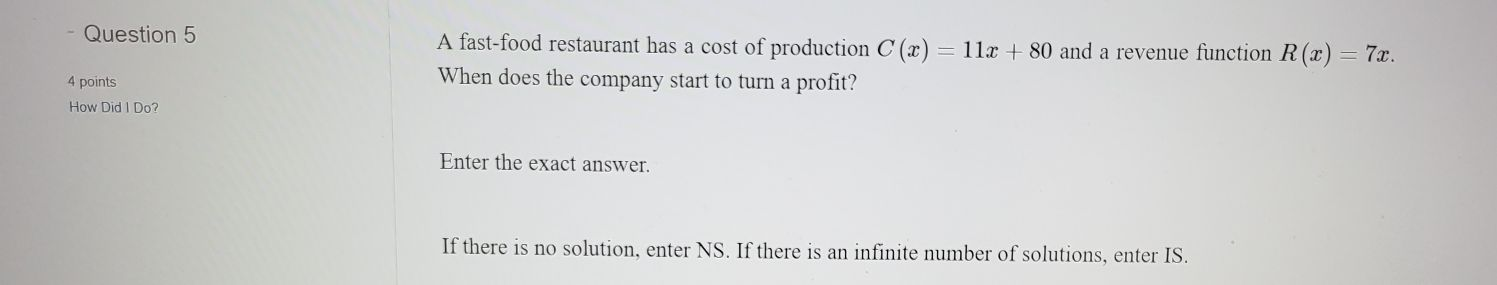### ¿Todavía tienes preguntas de matemáticas?

Pregunte a nuestros tutores expertos
Algebra
PreguntaA fast-food restaurant has a cost of production $$C ( x ) = 11 x + 80$$ and a revenue function $$R ( x ) = 7 x .$$

When does the company start to turn a profit? How Did I Do? Enter the exact answer. If there is no solution, enter NS. If there is an infinite number of solutions, enter IS.

$$p(x)= -4x- 80$$